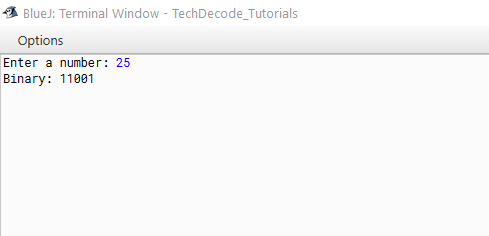# How to Convert Decimal to Binary in Java

If you have a foundation in computer science, broadening your technical knowledge is the finest thing you can do. However, your programming abilities are the most important. You must continue to polish them in order to become an effective programmer in the future.

Today let us know about How to Convert Decimal to Binary in Java but before all let us know about Binary and Decimal numbers a bit.  A binary number system is one of the four types of the number system. In computer applications, binary is zero (0) and one(1).

The binary numbers here are expressed in the base-2 numeral system. For example, (101) is a binary number. Decimal is a term that describes the base-10 number system, probably the most commonly used number system. The decimal number system consists of ten single-digit numbers: 0, 1, 2, 3, 4, 5, 6, 7, 8, and 9. The number after 9 is 10.

## What’s the Approach?

• Import the `java.util` package.

• Create the `Main` Function.

• Declare a `Scanner` Class.

• Accept the user `input` in decimal format.

• Create an `While loop` until the decimal number is `equal to zero.`

• The` binary number` is created by the formula.

• After each `iteration`, the number itself is changed to half of it.

• Print the `binary` number.

Also Read:- How to Convert String to Char in Java

### Java Program to Convert Decimal to Binary.

```/*
* TechDecode Tutorials
*
* How to Convert Decimalto Binary
*
*/
import java.util.*;
public class Decimal_To_Binary
{
public static void main(String[] args)
{
//Scanner Class
Scanner in= new Scanner(System.in);
int i=1;
int bin=0;
int rem;
//Enter the decimal number
System.out.print("Enter a number: ");
int dec = in.nextInt();

//Divide by 2 until number reduces to 0
while(dec!=0){
rem = dec%2;
bin += i*rem;
dec = dec/2;
i=i*10;
}

//Output the binary number
System.out.println("Binary: "+ bin);
}
}
```

Output:-